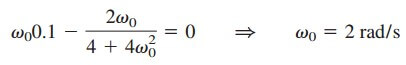# Parallel Resonance Circuit with Parallel RLC FormulaAfter learning about series resonance, we will move on to the parallel resonance.

Contents

This kind of resonance occurs when a circuit has an inductor and a capacitor connected together in parallel.

## Parallel Resonance

The parallel RLC circuit in Figure.(1) is the dual of the series RLC circuit.

or

Resonance occurs when the imaginary part of Y is zero,

or

which is the same as the equations for the series resonant circuit. The voltage |V| is sketched in Figure.(2) as a function of frequency.

Notice that at resonance, the parallel LC combination acts as an open circuit, so that the entire currents flow through R. Also, the inductor and capacitor current can be much more than the source current at resonance.

We exploit the duality between the series resonance circuit and Figure.(1) by comparing Equation.(2) with Equation.(3). By replacing R, L, and C in the expressions for the series circuit with 1/R, 1/C, and 1/L respectively, we obtain for the parallel circuit

Using Equations.(5) and (7), we can express the half-power frequencies in terms of the quality factor. The result is

Again, for high-Q circuits (Q 10)

Table.(1) presents a summary of the characteristics of the series and parallel resonant circuits. Besides the series and parallel RLC considered here, other resonant circuits exist.

## Parallel Resonance Examples

Let us review the parallel resonance examples below:

### Parallel Resonance Example 1

In the parallel RLC circuit in Figure.(3), let R = 8 kΩ, L = 0.2 mH, and C = 8 µF. (a) Calculate ω0, Q, and B. (b) Find ω1 and ω2. (c) Determine the power dissipated at ω0, ω1, and ω2.

Solution:
(a)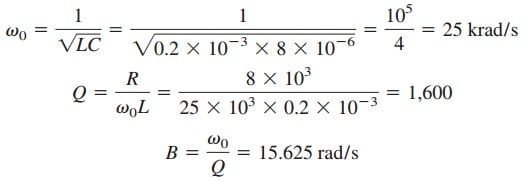(b) Due to the high value of Q, we can regard this as a high-Q circuit.
Hence,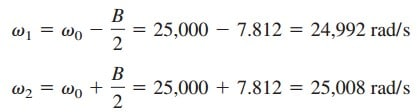(c) At ω = ω0, Y = 1/R or Z = R = 8 kΩ. ThenSince the entire current flows through R at resonance, the average power dissipated at ω = ω0 is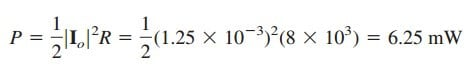or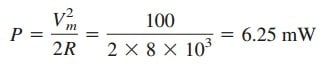At ω = ω1, ω2,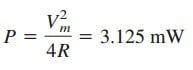### Parallel Resonance Example 2

Determine the resonant frequency of the circuit in Figure.(4).